Author: Oscar Cronquist Article last updated on February 22, 2021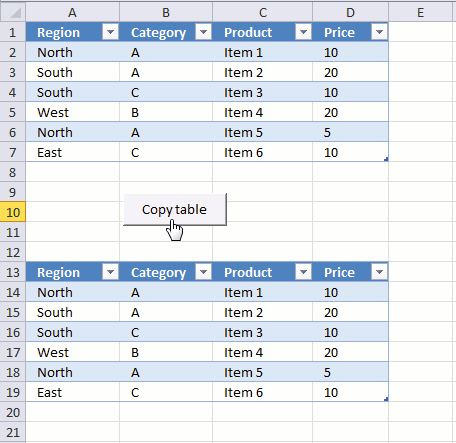The image above demonstrates a macro linked to a button. Press with left mouse button on the button and the macro runs. It copies the Excel table in cell range A1:D7 to cell range A13:D19.

## 1. How to copy an Excel Table programmatically?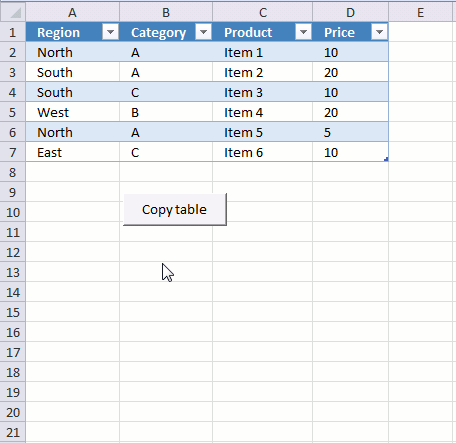The animated image above shows what happens when you press with left mouse button on the button. The VBA code below copies Table1 (Excel Table) to cell range A13 and adjacent cells.

```Sub CopyTable()
Range("Table1[#All]").Copy Destination:=Worksheets("2010").Range("A13")
End Sub```

Documentation: Range | Copy

## 2. How to copy the contents of a filtered Excel Table programmatically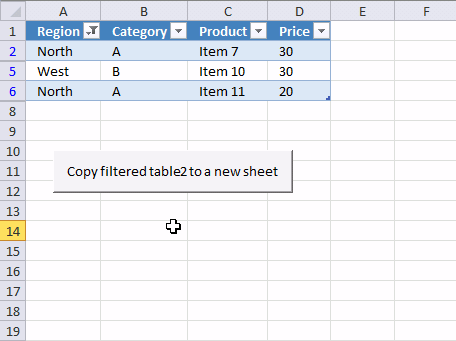The macro above works great if there are no filters applied to the table. If there were filters applied, all data would have been copied anyway.

So how do we solve that problem? This macro checks if each row in Table1 is visible. If it is, it is copied to a new sheet.

```'Name macro
Sub CopyFilteredTable()

'Dimension variables and declare datatypes
Dim rng As Range
Dim WS As Worksheet

'Go through rows in Table2
For Each Row In Range("Table2[#All]").Rows

'Check if row is visible
If Row.EntireRow.Hidden = False Then

'The SET statement allows you to save an object reference to a variable, the image above demonstrates a macro that assigns a range reference to a range object.
If rng Is Nothing Then Set rng = Row

'Returns the union of two or more ranges.
Set rng = Union(Row, rng)
End If

'Continue with next row
Next Row

'Create a new worksheet

'Copy rng to cell A1 in worksheet WS
rng.Copy Destination:=WS.Range("A1")
End Sub
```

Documentation: Range | Rows | If | Row | EntireRow | Hidden | Nothing | Set | Union | Sheets.add | copy | For Next | For Each Next  |

## 3. How to copy the contents of multiple filtered Excel tables to a new worksheetThe following macro iterates through each sheet in the current workbook and looks for Excel tables. It copies all visible values from every excel table to a new sheet.

This is handy if you have many excel tables in a workbook and you want to merge all filtered values from all tables to a new sheet.

```'Name macro
Sub CopyFilteredTables()

'Dimension variables and declare datatypes
Dim WS As Worksheet
Dim WSN As Worksheet
Dim tbl As ListObject
Dim rng As Range

'The SET statement allows you to save an object reference to a variable, the image above demonstrates a macro that assigns a range reference to a range object.

'Go through each worksheet in active workbook
For Each WS In Worksheets

'Go through Excel Tables in active worksheet
For Each tbl In WS.ListObjects

'Clear rng object
Set rng = Nothing

'Go through rows in Excel Table
For Each Row In tbl.DataBodyRange.Rows

'Check if row is hidden
If Row.EntireRow.Hidden = False Then

'Make sure rng object i nothing and then save row to rng
If rng Is Nothing Then Set rng = Row

'Returns the union of two or more ranges.
Set rng = Union(Row, rng)
End If

'Continue with next row in Excel Table
Next Row

'Save number of first non empty row to variable Lrow
Lrow = WSN.Range("A" & Rows.Count).End(xlUp).Row

'Check that number is larger than 1 and if so add 1 to variable Lrow
If Lrow > 1 Then Lrow = Lrow + 1

'Copy row
rng.Copy Destination:=WSN.Range("A" & Lrow)

'Continue with next Excel Table
Next tbl

'Continue with next worksheet
Next WS
End Sub
```

Documentation: Union | Nothing | If | copy | Row | EntireRow  | Hidden | Set | ListObjects |

## 4. How to apply a filter to all Excel Tables in a workbookThe image above demonstrates a macro that applies the same filter to all Excel Tables in the workbook. This is useful if they all share the same Table header names and you want to save time not having to manually manipulate each Excel Table.

Cell range A1:D1 contains header names and cells below contain filter conditions.

Instructions

1. Type the corresponding header, make sure you spell it right.
2. Type the critera below each header
3. Select the criteria with your mouse
4. Run macro ApplyFilterToTable
```'Name macro
Sub ApplyFilterToTable()

'Dimension variables and declare datatypes
Dim filters As Range
Dim flV() As Variant

'The SET statement allows you to save an object reference to a variable, the image above demonstrates a macro that assigns a range reference to a range object.
Set fltrs = Selection

'Redimension variable
ReDim flV(0 To Selection.Rows.CountLarge - 2)

'Cycle through all sheets in active workbook
For Each WS In Worksheets
'Cycle through all excel tables in sheet
For Each tbl In WS.ListObjects
For ct = 1 To tbl.DataBodyRange.Columns.Count
For cf = 1 To fltrs.Columns.Count
'Build array with filter values
j = 0
For i = LBound(flV) To UBound(flV)
If fltrs.Cells(i + 2, cf) <> "" Then
flV(i) = CStr(fltrs.Cells(i + 2, cf))
Else
flV(i) = ""
j = j + 1
End If
Next i
If tbl.Range.Cells(1, ct) = fltrs.Cells(1, cf) Then
'Clear filter
tbl.Range.AutoFilter Field:=ct
'Apply new filter
If UBound(flV) <> j - 1 Then
tbl.Range.AutoFilter Field:=ct, Criteria1:=flV, Operator:=xlFilterValues
End If
End If
Next cf
Next ct
Next tbl
Next WS
End Sub
```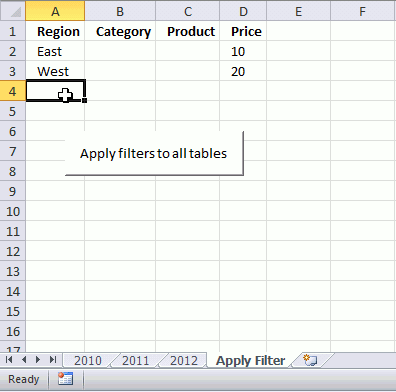It cycles through all excel tables in a workbook and applies the filter you have selected on a sheet.

## 5. How to apply filters to all Excel tables and copy the result to a new sheet

So if you combine macro CopyFilteredTables and ApplyFilterToTable you can quickly apply multiple filters to all excel tables and then copy the filtered values from all excel tables to a new sheet. That saves you a lot of time.

Instructions

1. Select the criteria. You also need to specify the corresponding header, make sure you spell it right.
2. Run macro ApplyFilterToTable
3. A new sheet is created and populated with values from all filtered
```Sub ApplyCopy()

'Start macro named ApplyFilterToTable
ApplyFilterToTable

'Start macro named CopyFilteredTables
CopyFilteredTables

End Sub
```

## 6. How to clear all filters from all Excel Tables in the active workbook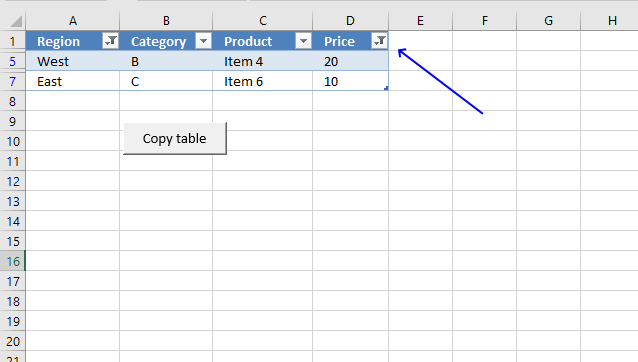The image above shows an Excel Table with a filter applied, you can tell the Excel Table is filtered by the button next to the Table header name. The image below shows the same Excel Table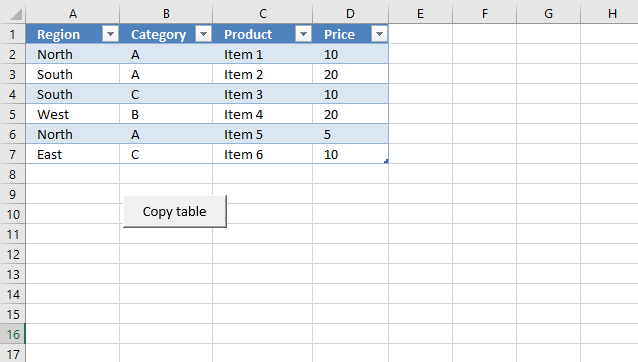The macro below removes all filters in all Excel Tables in the active workbook.

```'Name macro
Sub ClearFiltersAllTables()

'Go through all worksheets
For Each WS In Worksheets

'Got through all Excel Tables in Worksheet
For Each tbl In WS.ListObjects

'Go through all columns
For ct = 1 To tbl.DataBodyRange.Columns.Count

'Remove filter
tbl.Range.AutoFilter Field:=ct

'Continue with next column
Next ct

'Continue with next Excel Table
Next tbl
Next WS
End Sub
```

Documentation: AutoFilter | DataBodyRange | ListObjects | Worksheets

## 7. Where to put code?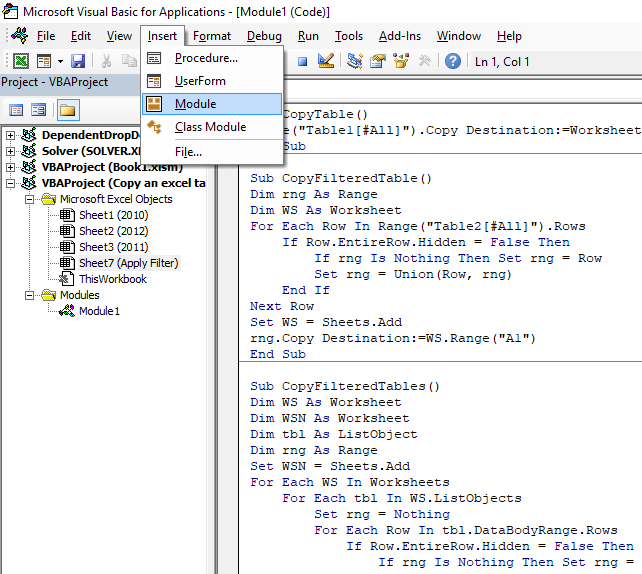1. Press Alt + F11 to open the Visual Basic Editor (VBE).
2. Press with left mouse button on "Insert" on the menu.
3. Press with left mouse button on Module to insert a new module to your workbook.
4. Copy VBA code.
5. Paste VBA code to the window, see image above.